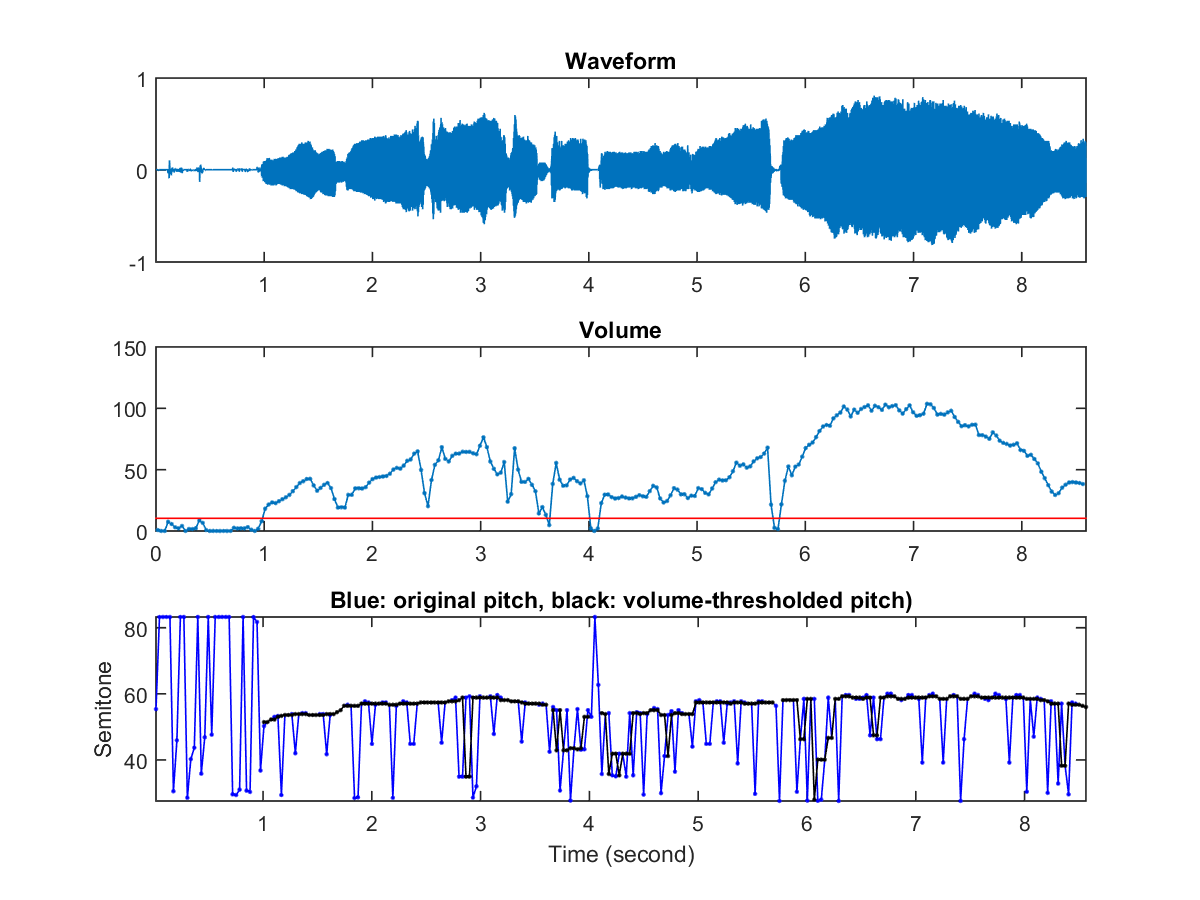## 7-8 Postprocessing: Smoothing

After we have obtained the pitch from a frame-based approach, it is advisable to perform postprocessing, including

• Smoothing: To smooth the pitch curve such that abrupt-changing pitch are removed.
• Interpolation: To increase the precision of the pitch

One commonly used technique for smoothing the pitch is the median filter, with an order of an odd small intergers, such as 3 or 5. The following example demonstrate the use of a median filter of order 5 to smooth the pitch:

Example 1: ptWithMedianFilter01.mwaveFile='soo.wav'; opt=pitchTrackBasic('defaultOpt'); opt.frame2pitchOpt.method=2; opt.medianFilterOrder=5; showPlot=1; pitch=pitchTrackBasic(waveFile, opt, showPlot);As you can see, the original pitch is full of abrupt-changing noise. After applying a median filter of order 5, some of the noise is correctly replaced with reasonable values. However, for a longer sequence of consecutive error pitch, we still need to rely on other dynamic-programming-based methods to fix them.

To increase the precision of the identified frame-based pitch, we usually apply parabolic interpolation around the maximum of the PDF. In particular, if $n_0$ if the maximizing index of a PDF, then we can fit a pababola that passes three points $(n_0-1, PDF(n_0-1))$, $(n_0, PDF(n_0))$, and $(n_0+1, PDF(n_0+1))$, and then use the maxmizing position of this parabola to compute the pitch. Here is an example:

Example 2: ptWithParabolicFit01.mwaveFile='soo.wav'; opt=pitchTrackBasic('defaultOpt'); pitch1=pitchTrackBasic(waveFile, opt); opt.frame2pitchOpt.useParabolicFit=1; pitch2=pitchTrackBasic(waveFile, opt); subplot(211); plot(1:length(pitch1), pitch1, 1:length(pitch2), pitch2); legend('Original pitch', 'Finetuned pitch with parabolic fit'); subplot(212); plot(1:length(pitch1), pitch1-pitch2); title('Pitch difference');

Audio Signal Processing and Recognition (音訊處理與辨識)